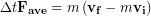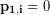# 9.11: Collisions

$$\newcommand{\vecs}{\overset { \rightharpoonup} {\mathbf{#1}} }$$ $$\newcommand{\vecd}{\overset{-\!-\!\rightharpoonup}{\vphantom{a}\smash {#1}}}$$$$\newcommand{\id}{\mathrm{id}}$$ $$\newcommand{\Span}{\mathrm{span}}$$ $$\newcommand{\kernel}{\mathrm{null}\,}$$ $$\newcommand{\range}{\mathrm{range}\,}$$ $$\newcommand{\RealPart}{\mathrm{Re}}$$ $$\newcommand{\ImaginaryPart}{\mathrm{Im}}$$ $$\newcommand{\Argument}{\mathrm{Arg}}$$ $$\newcommand{\norm}{\| #1 \|}$$ $$\newcommand{\inner}{\langle #1, #2 \rangle}$$ $$\newcommand{\Span}{\mathrm{span}}$$ $$\newcommand{\id}{\mathrm{id}}$$ $$\newcommand{\Span}{\mathrm{span}}$$ $$\newcommand{\kernel}{\mathrm{null}\,}$$ $$\newcommand{\range}{\mathrm{range}\,}$$ $$\newcommand{\RealPart}{\mathrm{Re}}$$ $$\newcommand{\ImaginaryPart}{\mathrm{Im}}$$ $$\newcommand{\Argument}{\mathrm{Arg}}$$ $$\newcommand{\norm}{\| #1 \|}$$ $$\newcommand{\inner}{\langle #1, #2 \rangle}$$ $$\newcommand{\Span}{\mathrm{span}}$$$$\newcommand{\AA}{\unicode[.8,0]{x212B}}$$

We can extend the concept of impulse introduced in the previous chapter to analyze forces and changes in velocity experienced by multiple objects during a collision. For example, we could determine the final velocity of two cars after a rear-end collision like this one:A YouTube element has been excluded from this version of the text. You can view it online here: openoregon.pressbooks.pub/bodyphysics/?p=1049

Let’s analyze this specific collision seen in the video. We start with our definition for impulse:

(1)## Momentum

Mass times velocity () is known as the momentum(p) , or. The change in momentum (Δp) is defined the same way as any other change, final momentum minus the initial momentum:. We can use these definitions to write the impulse-momentum theorem.

(2)Equivalently:

(3)## Conservation of Momentum

The impulse-momentum theorem indicates that if the average force acting on single object or a system of objects is zero, then the momentum of the object or system of objects is constant (conserved). The previous statement is known as the Principle of Conservation of Momentum. The related Law of Conservation of Momentum states that the combined total momentum of all objects in a system must be the same immediately before and immediately after a collision. We can treat both cars in our example collision as a single system as long as we account for the initial momentum and final momentum of both cars.

The initial momentum of the first (stopped) car was zero:. The momentum of the second car was:. Therefore the total initial momentum wasThe cars lock together immediately after the collision and only separate later so they have the same final velocity immediately after the collision, we’ll call it. Sticky collisions like this are known as perfectly inelastic collisions and for such collisions we can treat the objects moving together as a single object that has their combined total mass. The final momentum is then:Conserving momentum during the collision tells us to set the initial and final momenta equal:If we want to solve this perfectly inelastic collision equation for the final velocity we divide by the combined mass:We can look up some data on the cars and find that the length of the Jeep is 3.8 m, the mass of the jeep is about 1500 kg and a small sports car mass is about 1000 kg.  Now let’s estimate some numbers from the video (a quick method is to use the slo-mo feature on a smartphone to film the video as it plays with a running stopwatch also visible in the frame). We see the Jeep covers at least two of its own lengths in about 0.4 s so calling its direction the positive, its initial velocity will be:We are ready to calculate the final velocity:This is an interesting result, but what’s really cool is that if we estimate the collision interval we can reapply the impulse-momentum theorem to calculate the average force applied to each car. From the video the collision time appears to be about 0.5 s. Let’s do the Jeep first, just to think ahead, the force on the jeep should be in the negative direction based on our choice of backward and negative.

(4)We known from Newton's Third Law that the -23,000 N force on the Jeep from the car is paired with a 23,000 N force felt by the car from the Jeep. That’s over 5,000 lbs of force on each vehicle.

#### Reinforcement Exercise

A 48 kg soccer player running 5 m/s and watching the ball in the air collides with another player of mass 68 kg running at 2 m/s in the other direction and they tangle together before falling. What is the velocity of the pair immediately after the collision, before hitting the ground?

This page titled 9.11: Collisions is shared under a CC BY-NC-SA 4.0 license and was authored, remixed, and/or curated by Lawrence Davis (OpenOregon) via source content that was edited to the style and standards of the LibreTexts platform; a detailed edit history is available upon request.